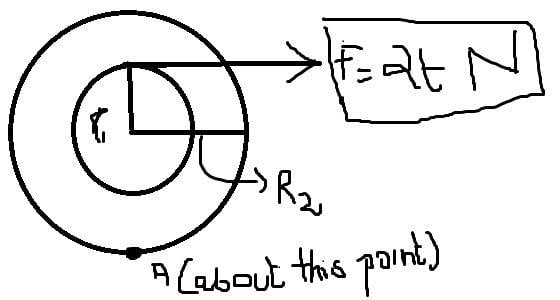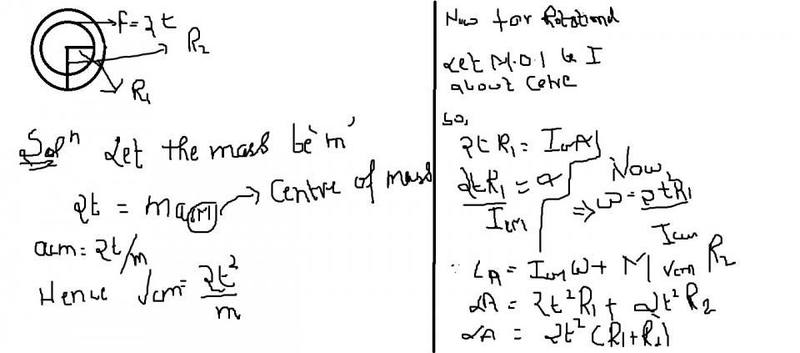# Rotational: angular momentum

• Yash123

## Homework Statementa spool of radius R1 and R2 (R2>R1) is kept on hortizontal surface. A force f= 2t N (where t is time ) acts on the inner radius tagentially find the angular momentum of the system about the bottomost point of the spool.

v=u+at
W=Wi+alpha(t)
L=IW+mvr

## The Attempt at a SolutionWhere is the pool fixed at the center or at the indicated point?

Where is the pool fixed at the center or at the indicated point?
It's not fixed ...it's on the floor free to rotate and translate

Nonetheless, could you give me the relation between ## \vec v## and ##\vec a##, and that between ##\vec α## and ##\vec ω##?

Nonetheless, could you give me the relation between ## \vec v## and ##\vec a##, and that between ##\vec α## and ##\vec ω##?

Nonetheless, could you give me the relation between ## \vec v## and ##\vec a##, and that between ##\vec α## and ##\vec ω##?
I solved it just used the wrong equations ...!

It's not fixed ...it's on the floor free to rotate and translate
but can you please solve iit jee paper 2 2016 rbd question

## Homework Statement

View attachment 107579
a spool of radius R1 and R2 (R2>R1) is kept on hortizontal surface. A force f= 2t N (where t is time ) acts on the inner radius tagentially find the angular momentum of the system about the bottomost point of the spool.

v=u+at
W=Wi+alpha(t)
L=IW+mvr

## The Attempt at a Solution

View attachment 107580
In your calculation of the linear acceleration you have ignored friction at the ground. Not sure if you did the same with the moments.
Please do not post working as images. Use images for diagrams and textbook extracts. Take the trouble to type in your algebra.

In your calculation of the linear acceleration you have ignored friction at the ground. Not sure if you did the same with the moments.
Please do not post working as images. Use images for diagrams and textbook extracts. Take the trouble to type in your algebra.
I solved it

You say F = 2t what about direction? Then you want angular momentum at what time. If time varying force is acting neither the momentum nor angular momentum will be constant.

•Yash123
You say F = 2t what about direction? Then you want angular momentum at what time. If time varying force is acting neither the momentum nor angular momentum will be constant.
Thanks for the reply but I solved it